Basic Electrical Concepts

# Basic Electrical Concepts | Electrical Engineering SSC JE (Technical) - Electrical Engineering (EE)

 1 Crore+ students have signed up on EduRev. Have you?

1. Electric Current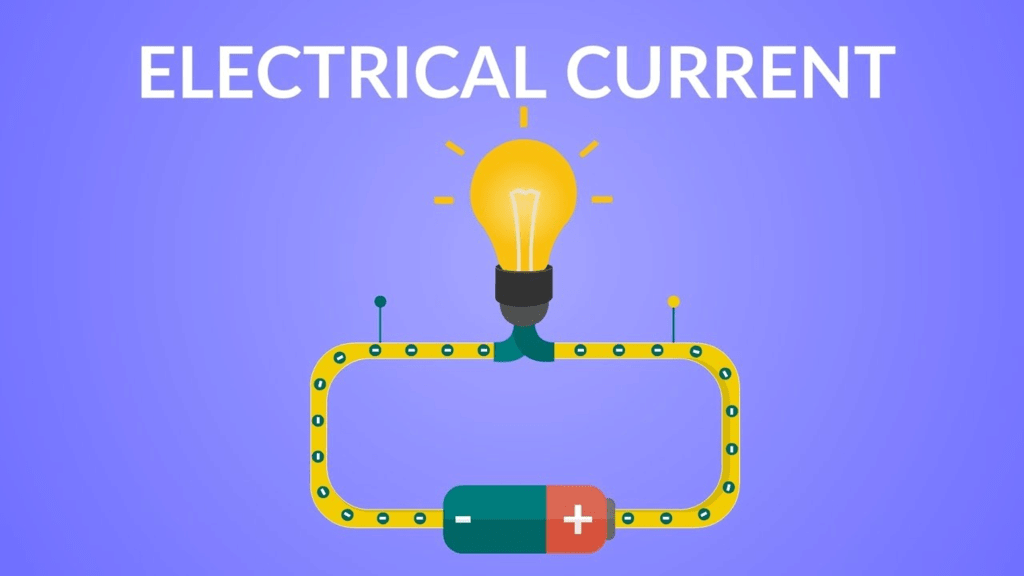The rate of flow of electric charge through any point of the circuit is called electric current. Its unit is ampere.

2. Coulomb's Law

According to this law, the force of attraction or repulsion between stationary point charges is directly proportional to the product of the charges and inversely proportional to the square of the distance between them.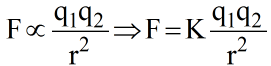Where K = 9 X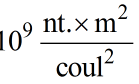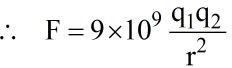Newton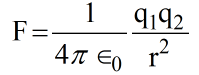3. Electric Field

It is the region surrounding an electric charge or group of charges, in which another charge experiences a force of attraction or repulsion.

4. Electric Lines of Forces

• An electric lines of force field along which a free isolated unit positive charge moves.
• Electric lines of force start from the positive charge and end to the negative charge.
• No two lines of force can intersect each other because if they do so, then at the point of intersection two tangents can be drawn which would mean two directions of force at that point which is impossible.
• These lines have a tendency to separate from each other in the direction perpendicular to their length.
• Lines of force of uniform field are parallel.
• Lines of force leaves the surface of the conductor normally.

5. Electric Flux

• It is defined as that total number of lines of force passing normally through a curved surface placed in the field or the dot product of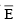and normal.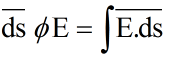1 unit = Volt-meter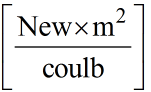6. Ohm's Law

• According to this law if there is no charge the physical state of conductor (Such as temp.) then the current flowing through the conductor is directly proportional to the voltage applied i, e.
I α V .
I =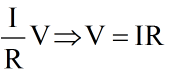• A graph between applied voltage and current is a straight line shows that it follows ohm's law.
• Ohm's law is valid for metallic conductors only.

7. Resistance

It is property of: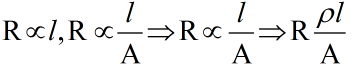ρ  = specific resistance or resistivity.

Note: The reciprocal of resistivity is called conductivity.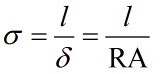(Ohm – m)–1 = mho/meter.

Colour Code for Resistances:

• These resistances are usually carbon resistances and whose code is used to indicate the value of resistance. 
• A carbon resistance has usually 4 concentric rings or bands A, B, C, D of different colours.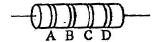• The colour of first two bands A and B indicate the 1st two significant figures of resistance in ohms & those of 3rd band C indicate the decimal multiplies. The 4th band D (Which is either silver or gold) tells the tolerance. 
• Sometimes only 3 colours band is there (A, B and C).

NOTE: B. ROY Great Britain Very Good Wife.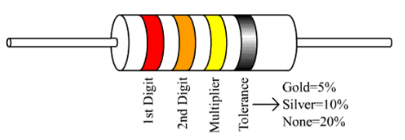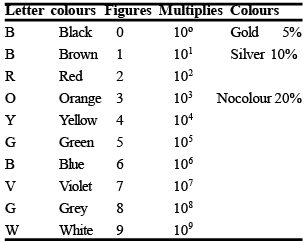8. Electric Potential 

• The electric potential at a point in an electric field is the ratio of the work done in bringing a test charge from infinity to that point to the magnitude of the test change. 
• If the work done in moving a test charge q0 from infinity to that point against the field is W, then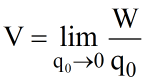Rate of charge of potential w.r.t distance in called potential gradient.

10. Electric Potential Energy

• The electric potential energy of a system of charges is the work that has to be done in bringing these charges from infinity to near each other to form the system.
• The potential energy of a system of charges q1 and q2 separated by a distance 'r' is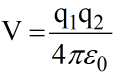11. Capacitor

An element in which energy is stored in the form of an electrostatic field is called a capacitor.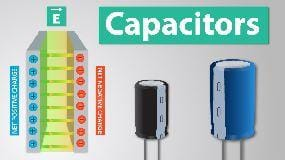12. Capacitance

Defined as the ratio of charge given to the plate of the capacitor to the potential difference produced between the plate.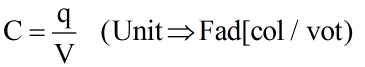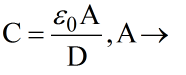Area of Plane

D → Distance between the planes.

0 = 8.85 x 10-12

0 = Permittivitty of free space.

Unit – c2N–1 M–2

For a capacitor, v ∝ q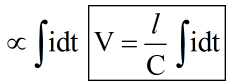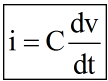Energy stored in a capacitor:

Energy(E) =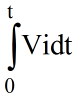=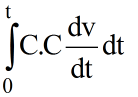= C.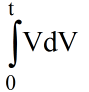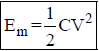13. Inductor

It is an element in which energy is stored in the form of an electromagnetic field (Unit  → Henry.)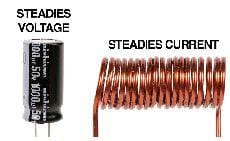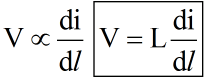Energy stored in Inductor: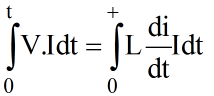= L.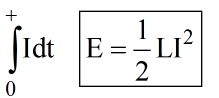14. Inductance

Inductance results from the fact that a flow of current produces a magnetic field, according to magnetic field along tends to induce a voltage (or current) in a wire according to Faraday's law.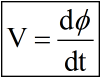V=Vm sin wt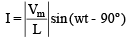show I lags by 90º R-L Load phasor :-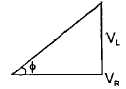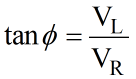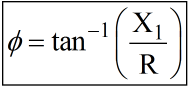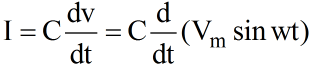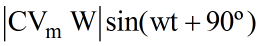(iii) For RC phases: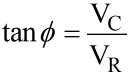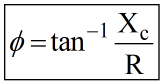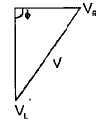Case 1: |VL| >  |Vc|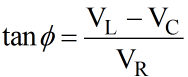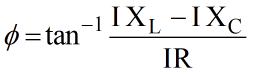Case 2: |VL| <  |Vc|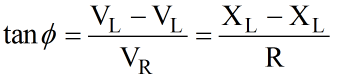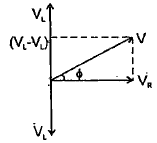Case 3: |VL| =  |Vc|

V = VR

Ø = 0

CosØ = 1(UPF)

Note:- Reactive power is consumed by energy storing demands.

The document Basic Electrical Concepts | Electrical Engineering SSC JE (Technical) - Electrical Engineering (EE) is a part of the Electrical Engineering (EE) Course Electrical Engineering SSC JE (Technical).
All you need of Electrical Engineering (EE) at this link: Electrical Engineering (EE)
FAQs on Basic Electrical Concepts | Electrical Engineering SSC JE (Technical) - Electrical Engineering (EE)
 1. What are the basic concepts of Electrical Engineering?Ans. Electrical Engineering (EE) is concerned with the study and application of electricity, electronics, and electromagnetism. The basic concepts of EE include: 1. Circuit Theory: It deals with the analysis of circuits and their behavior under different conditions. 2. Electromagnetic Theory: It deals with the study of the behavior of electromagnetic fields and their interactions with matter. 3. Electrical Machines: It deals with the study of electrical machines like generators, motors, transformers, and their working principles. 4. Power Systems: It deals with the generation, transmission, and distribution of electrical power. 5. Control Systems: It deals with the design and analysis of control systems that regulate and control the behavior of other systems.
 2. What is the difference between AC and DC current?Ans. AC (Alternating Current) and DC (Direct Current) are two types of electrical current. The main difference between them is the direction of the flow of electrons. In DC, the electrons flow in one direction, while in AC, the direction of the flow of electrons changes periodically. DC is used in batteries, electronic devices, and electric vehicles, while AC is used in power plants and homes. AC is more efficient for long-distance power transmission, while DC is more efficient for short-distance transmission.
 3. What are the units of electrical measurement?Ans. Electrical measurement is done in various units. Some of the important units are: 1. Voltage: Volt (V) 2. Current: Ampere (A) 3. Resistance: Ohm (Ω) 4. Power: Watt (W) 5. Energy: Joule (J) 6. Frequency: Hertz (Hz) 7. Capacitance: Farad (F) 8. Inductance: Henry (H) 9. Conductance: Siemens (S)
 4. What is the significance of Ohm's Law in Electrical Engineering?Ans. Ohm's Law is one of the most fundamental laws in electrical engineering. It states that the current flowing through a conductor is directly proportional to the voltage applied across it and inversely proportional to its resistance. Mathematically, Ohm's Law can be expressed as I = V/R, where I is the current, V is the voltage, and R is the resistance. This law is essential for designing and analyzing electrical circuits.
 5. What are the different types of electrical circuits?Ans. Electrical circuits can be broadly classified into two types: 1. Series Circuit: In a series circuit, the components are connected in a single path, and the current through each component is the same. 2. Parallel Circuit: In a parallel circuit, the components are connected in multiple paths, and the current is divided among them. There are also complex circuits that combine both series and parallel configurations. These circuits are commonly used in electronic devices, power systems, and control systems.

## Electrical Engineering SSC JE (Technical)

2 videos|75 docs|40 tests

## Electrical Engineering SSC JE (Technical)

2 videos|75 docs|40 tests

Track your progress, build streaks, highlight & save important lessons and more!(Scan QR code)

,

,

,

,

,

,

,

,

,

,

,

,

,

,

,

,

,

,

,

,

,

;